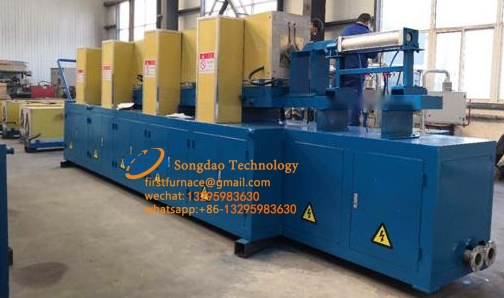### Induction furnace power estimation formula:

Induction furnace power estimation formula:

P=(C×G×T)/(0.24×t×∮)

Formula description: P—equipment power (KW); C—metal specific heat, of which the steel specific heat coefficient is 0.17;

G—the weight of the heated workpiece (kg); T—the heating temperature (℃); t—the work rhythm (seconds);

∮—The overall thermal efficiency of the equipment is generally 0.5-0.7, and about 0.4 for special-shaped parts.

For example: a forging factory has a forging blank of Φ60×150mm, the working cycle is 12 seconds/piece (including auxiliary time), and the initial forging temperature is 1200 °C. Then the calculation of the power of the GTR intermediate frequency electric furnace is required as follows: P=(0.17×3.3×1200)/(0.24×12×0.65)=359.61KW

According to the above calculation, GTR induction heating equipment with a rated power of 400KW can be configured.